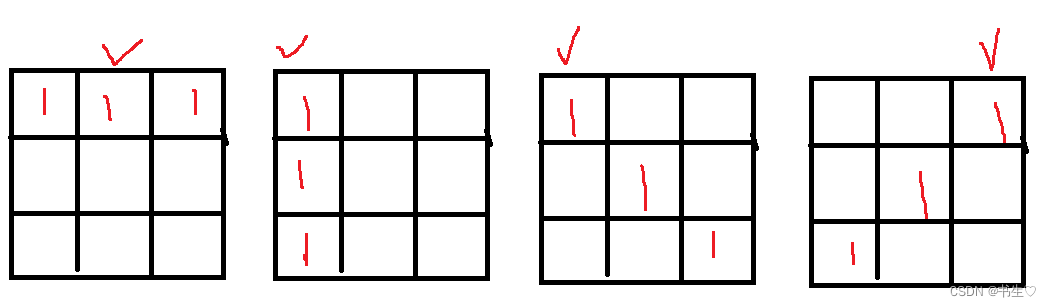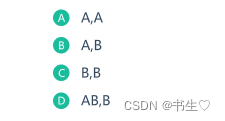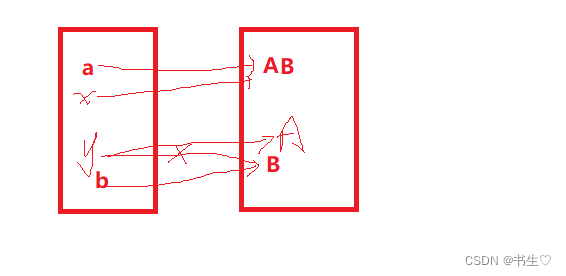## 强训之【井字棋和密码强度等级】

article2023/4/20 8:37:11/

• 1.井字棋
• 1.1题目
• 1.2思路讲解
• 1.3代码展示
• 2.密码强度判断
• 2.1题目
• 2.2思路讲解
• 2.3代码
• 3.选择题

# 1.井字棋

## 1.1题目

[[1,0,1],[1,-1,-1],[1,-1,0]]

## 1.2思路讲解## 1.3代码展示

``````import java.util.*;

public class Board {
public boolean checkWon(int[][] board) {
int sum = 0;
for (int i = 0; i < board.length; i++) {
sum=0;
for (int j = 0; j < board.length; j++) {
sum = sum + board[i][j];
}
if (sum == 3) {
return true;
}
}
for (int i = 0; i < board.length; i++) {
sum=0;
for (int j = 0; j < board.length; j++) {
sum = sum + board[j][i];
}
if (sum == 3) {
return true;
}
}

sum=0;
for (int i = 0; i < board.length; i++) {
sum = sum + board[i][i];
}
if (sum == 3) {
return true;
}
sum=0;
for (int i = 0; i < board.length; i++) {
sum = sum + board[i][board.length-1-i];
}
if (sum == 3) {
return true;
}
return false;
}
}
``````

# 2.密码强度判断

## 2.1题目

5 分: 小于等于4 个字符
10 分: 5 到7 字符
25 分: 大于等于8 个字符

0 分: 没有字母
10 分: 密码里的字母全都是小（大）写字母
20 分: 密码里的字母符合”大小写混合“

0 分: 没有数字
10 分: 1 个数字
20 分: 大于1 个数字

0 分: 没有符号
10 分: 1 个符号
25 分: 大于1 个符号

2 分: 字母和数字
3 分: 字母、数字和符号
5 分: 大小写字母、数字和符号

= 90: 非常安全
= 80: 安全（Secure）
= 70: 非常强
= 60: 强（Strong）
= 50: 一般（Average）
= 25: 弱（Weak）
= 0: 非常弱（Very_Weak）

VERY_SECURE
SECURE
VERY_STRONG
STRONG
AVERAGE
WEAK
VERY_WEAK

!"#\$%&'()*+,-./ (ASCII码：0x21~0x2F)
:;<=>?@ (ASCII码：0x3A~0x40)
[]^_` (ASCII码：0x5B~0x60)
{|}~ (ASCII码：0x7B~0x7E)

1 <= 字符串的长度<= 300

38\$@NoNoN

VERY_SECURE

Jl)M:+

AVERAGE

## 2.2思路讲解

1.我们先判断字符串的长度，很简单就不多说了
2.字母，我们判断条条件为，定义两个变量，count1和count2，如果出现小写字母（该下标的字母ASCII在‘a’-‘z’之间就是小写字母），count1++，反之如果出现大写字母（该下标的字母ASCII在‘A’-‘Z’之间就是大写字母），count2++.然后判断count1和count2是否为0，如果都为0，没有字母，有一个就全是小写/大写的，全都不为0，就是混合的字母。
3.数字，这个也是一样，判断字符是否处于‘0’-‘9’之间，有的话count3++,最后判断count3的数量
4一样判字符是否处于符号的ASCII之间，不过这个符号的区间不是连续的所以需要用上 ||，这个逻辑运算符，（ch[i] >= 0x21 && ch[i] <= 0x2F || ch[i] >= 0x3A && ch[i] <= 0x40|| ch[i] >= 0x5B && ch[i] <= 0x60 || ch[i] >= 0x7B && ch[i] <= 0x7E )
5.最后判断 都有哪些

## 2.3代码

``````import java.util.Scanner;

public class Main {

public static int score(String str) {
char[] ch = str.toCharArray();
int sum = 0;
int len = ch.length;
//计算长度
if (len <= 4) {
sum += 5;
} else if (len >= 5 && len <= 7) {
sum += 10;
} else {
sum += 25;
}
//计算数字
int count = 0;
for (int i = 0; i < len; i++) {
if (ch[i] >= '0' && ch[i] <= '9') {
count++;
}
}
if (count == 0) {
sum += 0;
} else if (count == 1) {
sum += 10;
} else {
sum += 20;
}
//计算字母
int count1 = 0;
int count2 = 0;
for (int i = 0; i < len; i++) {
if (ch[i] >= 'a' && ch[i] <= 'z' ) {
count1++;//小写字母
} else if (ch[i] >= 'A' && ch[i] <= 'Z') {
count2++;//大写的
}
}
if (count1 == 0 && count2 == 0) {
sum += 0;
} else if (count1 != 0 && count2 != 0 ) {
sum += 20;
} else {
sum += 10;
}

//计算符号
int count3 = 0;
for (int i = 0; i < len; i++) {
if (ch[i] >= 0x21 && ch[i] <= 0x2F || ch[i] >= 0x3A && ch[i] <= 0x40
|| ch[i] >= 0x5B && ch[i] <= 0x60  || ch[i] >= 0x7B && ch[i] <= 0x7E ) {
count3++;
}
}
if (count3 == 0) {
sum += 0;
} else if (count3 == 1) {
sum += 10;
} else {
sum += 25;
}
//奖励

if ((count1 > 0 && count2 > 0) && count > 0 && count3 > 0) {
sum += 5;
} else if (count > 0 && (count1 != 0 || count2 != 0) && count3 > 0) {
sum += 3;
} else if (count > 0 && (count1 != 0 || count2 != 0)) {
sum += 2;
}

return sum;

}
public static void main(String[] args) {
Scanner in = new Scanner(System.in);
String str = in.nextLine();
int sum = score(str);
if (sum >= 90) {
System.out.println("VERY_SECURE");
} else if (sum >= 80 && sum < 90) {
System.out.println("SECURE");
} else if (sum >= 70 && sum < 80) {
System.out.println("VERY_STRONG");
} else if (sum >= 60 && sum < 70) {
System.out.println("STRONG");
} else if (sum >= 50 && sum < 60) {
System.out.println("AVERAGE");
} else if (sum >= 25 && sum < 50) {
System.out.println("WEAK");
} else {
System.out.println("VERY_WEAK");
}

}
}
``````

# 3.选择题

1. 下列运算符合法的是（A
A &&
B <>
C if
D :=

<>这是这个是定义泛型的
if判断语句的关键字

2.下面代码运行结果是（C

``````public class Test{
try {
return a+b;
}
catch (Exception e) {
System.out.println("catch语句块");
}
finally{
System.out.println("finally语句块");
}
return 0;
}
public static void main(String argv[]){
Test test =new Test();
}
}
``````

A catch语句块 和是：43
B 编译异常
C finally语句块 和是：43
D 和是：43 finally语句块

3.下列Java代码中的变量a、b、c分别在内存的____存储区存放。（C
class A {
private String a = “aa”;
public boolean methodB() {
String b = “bb”;
final String c = “cc”;
}
}
A 堆区、堆区、堆区
B 堆区、栈区、堆区
C 堆区、栈区、栈区
D 堆区、堆区、栈区
E 静态区、栈区、堆区
F 静态区、栈区、栈区

1. 以下声明合法的是（B
A default String s
B public final static native int w( )
C abstract double d
D abstract final double hyperbolicCosine( )

default不是权限修饰符
final修饰的不能被重写，但是接口就是来被继承重写，所以不能一起使用

1. 在使用super 和this关键字时，以下描述正确的是（A
A 在子类构造方法中使用super（）显示调用父类的构造方法，super（）必须写在子类构造方法的第一
行，否则编译不通过
B super（）和this（）不一定要放在构造方法内第一行
C this（）和super（）可以同时出现在一个构造函数中
D this（）和super（）可以在static环境中使用，包括static方法和static语句块

6.下面代码的输出结果是什么？（D
public class ZeroTest {
public static void main(String[] args) {
try{
int i = 100 / 0;
System.out.print(i);
}catch(Exception e){
System.out.print(1);
throw new RuntimeException();
}finally{
System.out.print(2);
}
System.out.print(3);
}
}
A 3
B 123
C 1
D 12

7.关于下面代码片段叙述正确的是（C

byte b1=1,b2=2,b3,b6;
final byte b4=4,b5=6;
b6=b4+b5;
b3=(b1+b2);
System.out.println(b3+b6);
A 输出结果：13
B 语句：b6=b4+b5编译出错
C 语句：b3=b1+b2编译出错
D 运行期抛出异常

8.以下java程序代码，执行后的结果是（A
public class Test {
public static void main(String[] args) {
Object o = new Object() {
public boolean equals(Object obj) {
return true;
}
};
System.out.println(o.equals(“Fred”));
A Fred
B true
C 编译错误
D 运行时抛出异常
}
}

Object是所有类的父类，重写了equals方法，直接返回true。

9.执行以下程序后的输出结果是（D
public class Test {
public static void main(String[] args) {
StringBuffer a = new StringBuffer(“A”);
StringBuffer b = new StringBuffer(“B”);
operator(a, b);
System.out.println(a + “,” + b);
}
public static void operator(StringBuffer x, StringBuffer y) {
x.append(y); y = x;
}
}10.下面所示的java代码，运行时，会产生（）类型的异常（D
int Arry_a[] = new int;
System.out.println(Arry_a);

A ArithmeticException
B NullPointException
C IOException
D ArrayIndexOutOfBoundsException

### redis——使用

session缓存缓存更新方式删除缓存vs更新缓存缓存和数据库操作原子性缓存和数据库操作顺序结论 缓存问题缓存穿透缓存雪崩缓存击穿 全局唯一ID数据并发线程安全单体分布式redis分布式锁的问题 redis消息队列listpubsubstream 消息推送 session 问题&#xff1a;session存在tomca…

### nginx简单使用与配置

nginx简单使用与配置 Nginx 是一个高性能的HTTP和反向代理web服务器、一个邮件代理服务器&#xff0c;一个通用的 TCP/UDP 代理服务器。支持FastCGI、SSL、Virtual Host、URL Rewrite、Gzip等功能。并且支持很多第三方的模块扩展。 前端可以通过nginx实现以下功能&#xff1a…

### Linux--tty

Linux 终端(TTY) TTY 是 Teletype 或 Teletypewriter 的缩写&#xff0c;原来是指电传打字机&#xff0c;后来这种设备逐渐键盘和显示器取代。不管是电传打字机还是键盘显示器&#xff0c;都是作为计算机的终端设备存在的&#xff0c;所以 TTY 也泛指计算机的终端(terminal)设…

### 基于DSP+FPGA的多轴运动控制平台（一）硬件设计

2 实验平台总体方案与硬件设计 2.1.1 实验平台的功能需求分析 针对便于多轴运动控制技术的研究&#xff0c;培养此方面技术的人才&#xff0c;实验平台应能 对多轴运动实现高速高精度的控制效果&#xff0c;同时保证系统开放性和兼容多种算法及 参数的运行。 实验过程契合…

### wait 和 notify

wait 和 notify 7. wait 和 notify7.1 wait()方法7.2 notify()方法★★★wait和notify代码wait 需要搭配 synchronized 7.3 notifyAll()方法7.4 wait 和 sleep 的对比&#xff08;面试题&#xff09; 7. wait 和 notify 保证其他线程可以正常进行 由于线程之间是抢占式执行的, …

### 风电的Weibull分布及光电的Beta分布组合研究（Matlab代码实现）

&#x1f4a5;&#x1f4a5;&#x1f49e;&#x1f49e;欢迎来到本博客❤️❤️&#x1f4a5;&#x1f4a5; &#x1f3c6;博主优势&#xff1a;&#x1f31e;&#x1f31e;&#x1f31e;博客内容尽量做到思维缜密&#xff0c;逻辑清晰&#xff0c;为了方便读者。 ⛳️座右铭&a…

### OA系统的功能和作用是什么（OA系统百科）

OA系统的功能和作用是什么&#xff08;OA系统百科&#xff09;。OA系统是一种非常实用的企业内部管理系统&#xff0c;它可以帮助公司实现各项管理工作&#xff0c;可以说是整个公司和团队的纽带&#xff0c;有助于提高工作效率和管理水平。 具体来说&#xff0c;OA系统的作用…